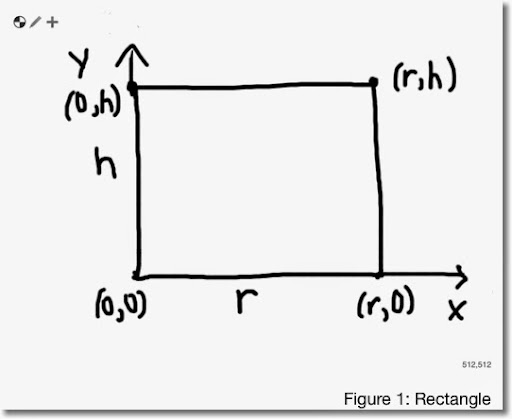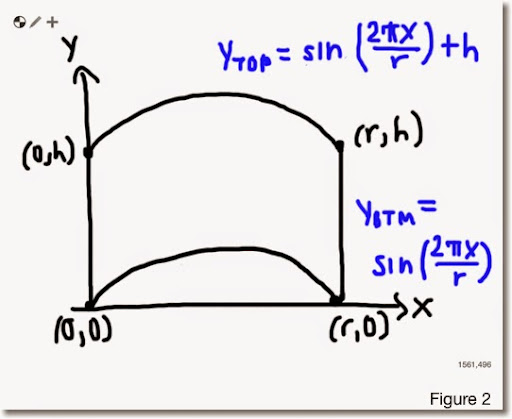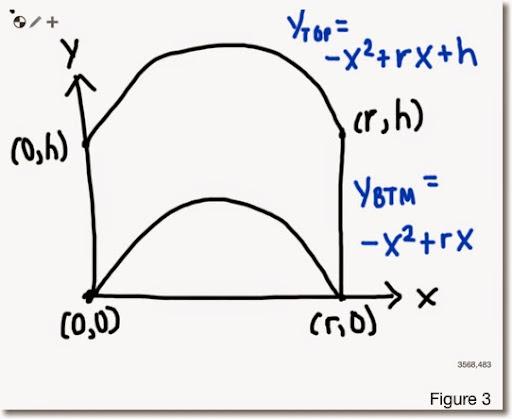## Friday, July 4, 2014

### Area: Interesting ResultsArea 1 (see figure 1 above)

This is a simple rectangle.

A = r * h

Easy, right? Suppose that two of the sides are not straight lines but sinusoidal curves, like in Figure 2 below.yTOP = sin(2 * π * x/r) + h
yBTM = sin(2 * π * x/r)

Left boundary: x = 0
Right boundary: x = r

Area:

r
∫ yTOP - yBTM dx
0

r
∫ sin(2 * π * x/r) + h - sin(2 * π * x/r) dx
0

r
∫ h dx
0

r * h

Hmmm. Let's try something with curves shaped parabolically, like in Figure 3.yTOP = -x^2 + r * x + h
yBTM = -x^2 + r * x

Left boundary: x = 0
Right boundary: x = r

Area:

r
∫ yTOP - yBTM dx
0

r
∫ -x^2 + r * x + h - (-x^2 + r * h) dx
0

r
∫ h dx
0

r * h

Interesting that the same result is obtained in all three cases, A = r * h.

Keep in mind that these are specific shapes. Who said math isn't fun? :)

Eddie

This blog is property of Edward Shore. 2014

#### 2 comments:

1.Great post. Lots of material in one tidy package. Much nourishment:)

2.I think this generalizes. If Ytop = f(x)+h and Ybottom=f(x) then the area is still r*h. This should work for any f(x) that's defined over the interval, even one that has no integral itself... I think....

### Swiss Micros DM16L: Advanced Boolean and Factorial (up to 20)

Swiss Micros DM16L:   Advanced Boolean and Factorial (up to 20) Introduction The program listing, for the Swiss Micros DM16L and Hewlett Pac...